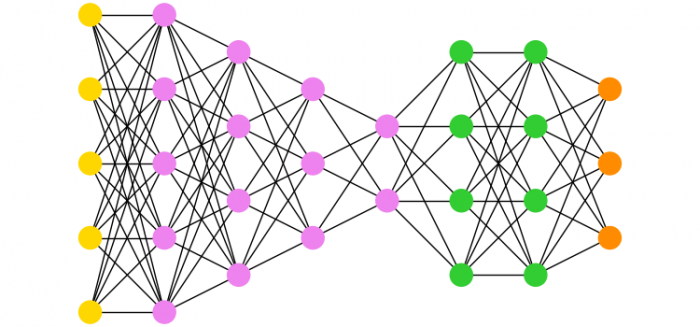# How to make multipartite graphs using networkx and Matplotlib?

To make multipartite graph in networkx, we can take the following steps −

• Set the figure size and adjust the padding between and around the subplots.

• Create a list of subset sizes and colors.

• Define a method for multilayered graph that could return a multilayered graph object.

• Set the color of the nodes.

• Position the nodes in layers of straight lines.

• Draw the graph G with Matplotlib.

• Set equal axis properties.

• To display the figure, use show() method.

## Example

import itertools
import matplotlib.pyplot as plt
import networkx as nx

plt.rcParams["figure.figsize"] = [7.50, 3.50]
plt.rcParams["figure.autolayout"] = True

subset_sizes = [5, 5, 4, 3, 2, 4, 4, 3]
subset_color = [
"gold",
"violet",
"violet",
"violet",
"violet",
"limegreen",
"limegreen",
"darkorange",
]

def multilayered_graph(*subset_sizes):
extents = nx.utils.pairwise(itertools.accumulate((0,) + subset_sizes))
layers = [range(start, end) for start, end in extents]
G = nx.Graph()
for (i, layer) in enumerate(layers):
for layer1, layer2 in nx.utils.pairwise(layers):
return G

G = multilayered_graph(*subset_sizes)
color = [subset_color[data["layer"]] for v, data in G.nodes(data=True)]
pos = nx.multipartite_layout(G, subset_key="layer")
nx.draw(G, pos, node_color=color, with_labels=False)

plt.axis("equal")

plt.show()

## Output